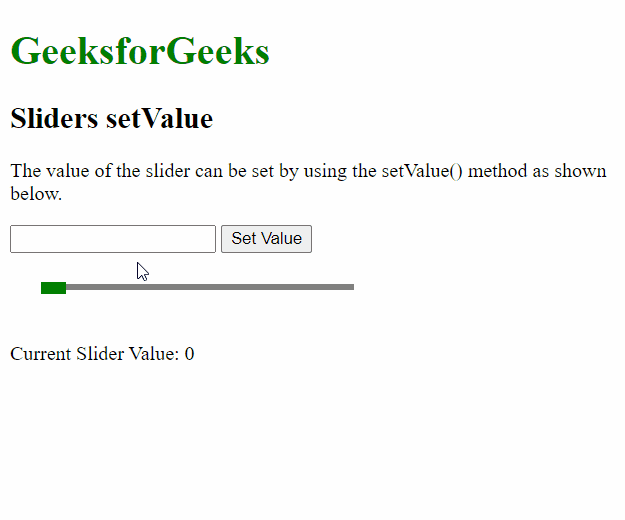# script.aculo.us Sliders setValue option

• Last Updated : 27 Nov, 2020

The script.aculo.us library is a cross-browser library that aims at improving the user interface of a website. The Slider controls are thin tracks that allow the user to input values. It is done by defining a range of values that can be selected by the user by dragging the handle to the appropriate value.

The Sliders setValue function is used to update the value of the slider to the given value. It will move the handle of the slider to the appropriate position of the slider track. The onChange() callback function is invoked whenever this method is used and can be used to track the change in value.

Hey geek! The constant emerging technologies in the world of web development always keeps the excitement for this subject through the roof. But before you tackle the big projects, we suggest you start by learning the basics. Kickstart your web development journey by learning JS concepts with our JavaScript Course. Now at it's lowest price ever!

Syntax:

`setValue( value, handleIndex )`

Parameters: This function has two parameters as mentioned above and described below:

• value: It is a number that will be used to update the value of the slider.
• handleIndex: It is used to define the index of the slider to be updated when using multiple sliders. It is an optional parameter. When this value is not passed, then the last used slider is updated.

Example:

 ```<``html``>``<``head``>``  ````  ``<``script` `type``=``"text/javascript"``          ``src``=``"prototype.js"``>``  ````  ``<``script` `type``=``"text/javascript"` `          ``src``=``"scriptaculous.js?load = slider"``>``  ```` ` `  ````  ``<``style` `type``=``"text/css"``>``    ``.track {``      ``width: 250px;``      ``background-color: gray;``      ``height: 5px;``      ``position: relative;``    ``}`` ` `    ``.track .handle {``      ``width: 20px;``      ``height: 10px;``      ``background-color: green;``      ``cursor: move;``      ``position: absolute;``      ``top: -2px;``    ``}`` ` `    ``.pad {``      ``padding: 25px;``    ``}``  ``````<``body``>``  ``<``p``>``  ``<``h1` `style``=``"color: green;"``>``    ``GeeksforGeeks``  ````  ``<``h2``>Sliders setValue``  ``<``p``>``    ``The value of the slider can be set``    ``by using the setValue() method ``    ``as shown below.``  ```` ` `  ``<``input` `type``=``"text"` `id``=``"val"``>``  ``<``button` `onclick``=``"setVal()"``>``    ``Set Value``  ```` ` `  ``<``div` `class``=``"pad"``>``    ``<``div` `id``=``"track-hor"` `class``=``"track"``>``      ``<``div` `id``=``"handle-hor"` `class``=``"handle"``>``      ````    ````  ````  ``<``p``>Current Slider Value: ``    ``<``span` `id``=``"out"``>0``  ```` ` `  ``<``script` `type``=``"text/javascript"``>`` ` `    ``// Initialize the slider``    ``let slider = new Control.Slider(``      ``'handle-hor',``      ``'track-hor', {``       ` `      ``// Define the range``      ``range: \$R(1, 100),`` ` `      ``onChange: function (v) {``        ``document.querySelector("#out")``                ``.textContent = v;``      ``}``    ``});`` ` `    ``function setVal() {`` ` `      ``// Get the value form the input box``      ``let val = ``          ``document.querySelector("#val").value;`` ` `      ``// Set the value of the slider``      ``// to the given value``      ``slider.setValue(val);``    ``}``  ```````

Output:My Personal Notes arrow_drop_up